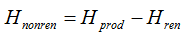# HOMER Pro 3.9

 Navigation: Glossary Nonrenewable Thermal ProductionType: Intermediate Variable Units: kWh/yr Symbol: Hnonren

The nonrenewable thermal production is the total amount of thermal energy produced annually by non-renewable thermal energy sources. HOMER uses the following equation to calculate the nonrenewable thermal production:where: Hprod = total thermal production [kWh] Hren

HOMER uses this value to calculate the renewable fraction.

See also

Renewable Fraction

Renewable Electrical Production

Total Thermal Production